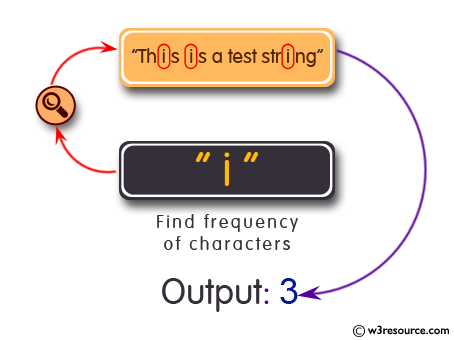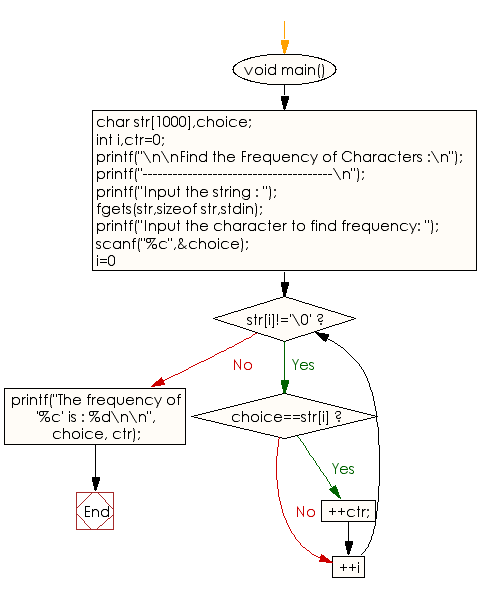﻿ C exercises: Find the Frequency of Characters - w3resource# C Programming: Find the Frequency of Characters

## C String: Exercise-18 with Solution

Write a program in C to Find the Frequency of Characters.Sample Solution:

C Code:

``````#include <stdio.h>
void main(){
char str,choice;
int i,ctr=0;

printf("\n\nFind the Frequency of Characters :\n");
printf("--------------------------------------\n");

printf("Input the string : ");
fgets(str,sizeof str,stdin);

printf("Input the character to find frequency: ");
scanf("%c",&choice);
for(i=0;str[i]!='\0';++i)
{
if(choice==str[i])
++ctr;
}
printf("The frequency of '%c' is : %d\n\n", choice, ctr);
}
```
```

Sample Output:

```Find the Frequency of Characters :
--------------------------------------
Input the string : This is a test string
Input the character to find frequency: i
The frequency of 'i' is : 3
```

Flowchart :C Programming Code Editor:

Improve this sample solution and post your code through Disqus.

What is the difficulty level of this exercise?

﻿

## C Programming: Tips of the Day

Is there a way to specify how many characters of a string to print out using printf()?

The basic way is:

`printf ("Here are the first 8 chars: %.8s\n", "A string that is more than 8 chars"); `

The other, often more useful, way is:

`printf ("Here are the first %d chars: %.*s\n", 8, 8, "A string that is more than 8 chars"); `

Here, you specify the length as an int argument to printf(), which treats the '*' in the format as a request to get the length from an argument.

You can also use the notation:

```printf ("Here are the first 8 chars: %*.*s\n",
8, 8, "A string that is more than 8 chars"); ```

This is also analogous to the "%8.8s" notation, but again allows you to specify the minimum and maximum lengths at runtime - more realistically in a scenario like:

`printf("Data: %*.*s Other info: %d\n", minlen, maxlen, string, info); `

The POSIX specification for printf() defines these mechanisms.

Ref: https://bit.ly/395Zj2j